Next: General interaction between macrosystems Up: Statistical thermodynamics Previous: Temperature

## Mechanical interaction between macrosystems

Let us now examine a purely mechanical interaction between macrostates, where one or more of the external parameters is modified, but there is no exchange of heat energy. Consider, for the sake of simplicity, a situation where only one external parameterof the system is free to vary. In general, the number of microstates accessible to the system when the overall energy lies betweenanddepends on the particular value of, so we can write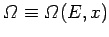.

Whenis changed by the amount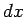, the energy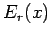of a given microstatechanges by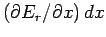. The number of states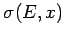whose energy is changed from a value less thanto a value greater thanwhen the parameter changes fromto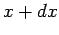is given by the number of microstates per unit energy range multiplied by the average shift in energy of the microstates. Hence,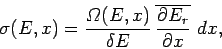(190)

where the mean value of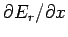is taken over all accessible microstates (i.e., all states where the energy lies betweenandand the external parameter takes the value). The above equation can also be written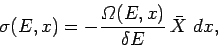(191)

where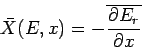(192)

is the mean generalized force conjugate to the external parameter(see Sect. 4.4).

Consider the total number of microstates betweenand. When the external parameter changes fromto, the number of states in this energy range changes by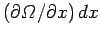. This change is due to the difference between the number of states which enter the range because their energy is changed from a value less thanto one greater thanand the number which leave because their energy is changed from a value less thanto one greater than. In symbols,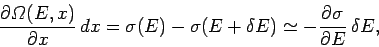(193)

which yields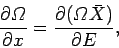(194)

where use has been made of Eq. (191). Dividing both sides by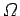gives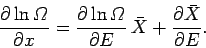(195)

However, according to the usual estimate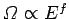, the first term on the right-hand side is of order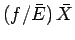, whereas the second term is only of order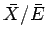. Clearly, for a macroscopic system with many degrees of freedom, the second term is utterly negligible, so we have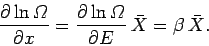(196)

When there are several external parameters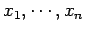, so that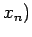, the above derivation is valid for each parameter taken in isolation. Thus,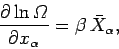(197)

where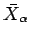is the mean generalized force conjugate to the parameter.Next: General interaction between macrosystems Up: Statistical thermodynamics Previous: Temperature
Richard Fitzpatrick 2006-02-02